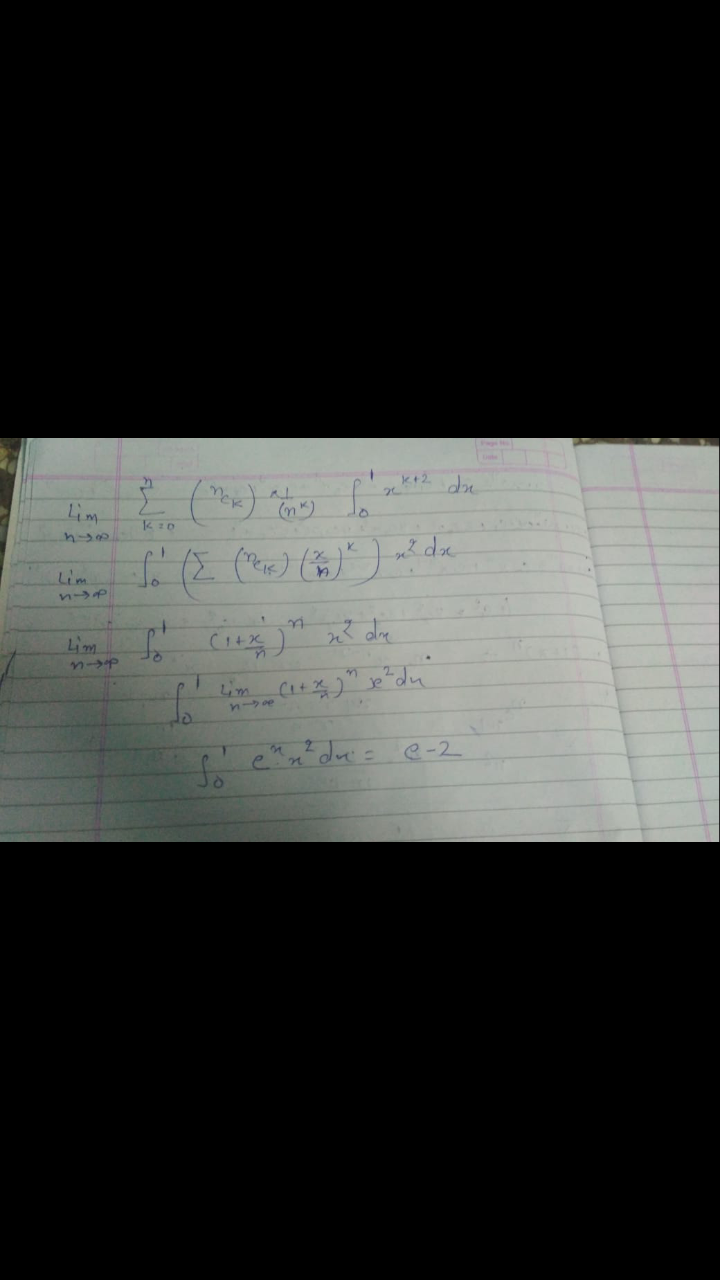# Thread to share Good Questions from Calculus which requires deep thinking

Is the answer 18 ? @Sourav_Dey sir ?

Yes @ Mayank ... share your approach !

First removed the x^2 from the denominator by substituting x^3 = t
So numerator is
\int^{1}_{0} lnx ln(1-x) dx
and denominator is
1 \over 9 \int^{0.5}_{0} lnx ln(1-x) dx
Now we can clearly see that the integral in numerator is twice that of in denominator
So we get 18

3 Likes
1 Like

Which package is this archive or revision or which ?

It's archive

@Sourav_Dey Sir, solution of this question please

Is it \sqrt 2 @Sourav_Dey Sir ?

No check again

The Integral is \frac{I_{n+1}}{I_{n}}
Limits from pi/4 to 3pi/4 integral of sin
Now we can use reduction formula maybe?
@Mayank_Chowdhary what did you get

i did the same and got an infinite series
something like
n(n-2)(n-4).../ (n+1)(n-1)(n-3).... the whole squared with a root 2 outside it

@Mayank_Chowdhary yes √2 is correct .. submit your solution

@Siddhant_Mudholkar which question solution do you ask me ?

got the solution sir @Sourav_Dey

I am also getting \color{brown}\sqrt{2}.

Yes Ur approach sir